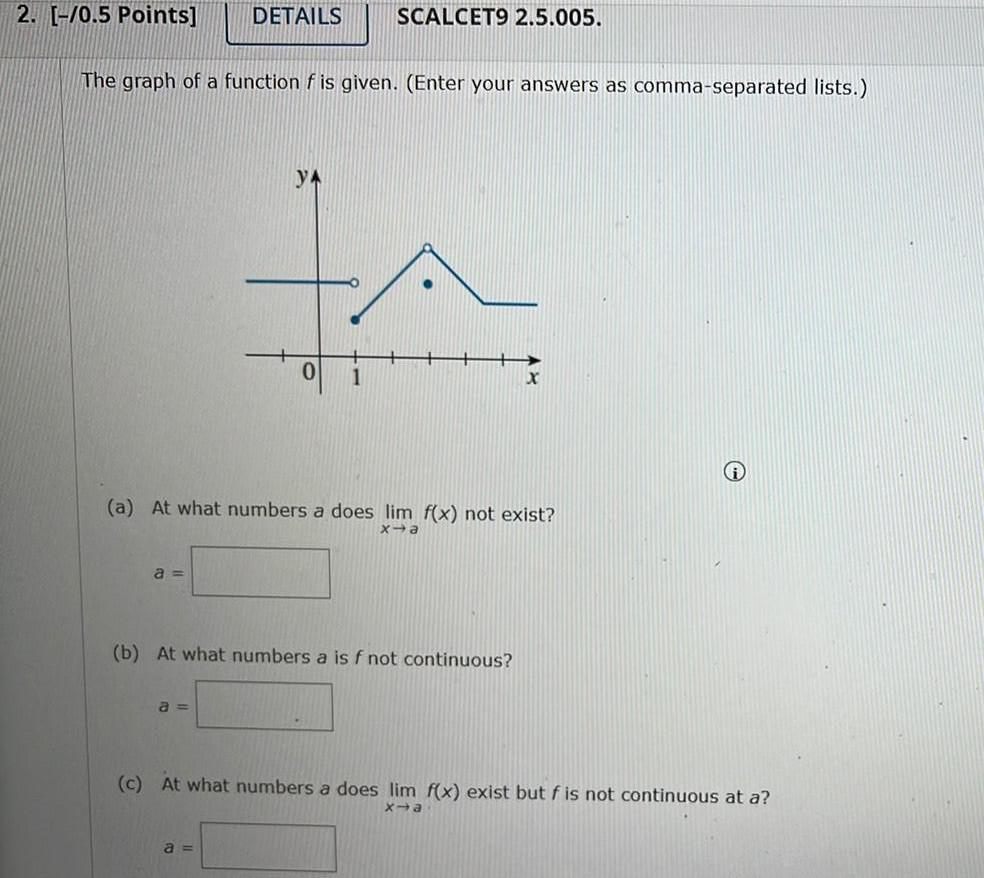Question:

# 2. [-70.5 Points] DETAILS SCALCET9 2.5.005. The graph of a function f is given. (Enter your answers as comma-separated lists.) 02. [-70.5 Points] DETAILS SCALCET9 2.5.005. The graph of a function f is given. (Enter your answers as comma-separated lists.) 0 1 (a) At what numbers a does lim f(x) not exist? xa a = (b) At what numbers a is f not continuous? a = (c) At what numbers a does lim f(x) exist but f is not continuous at a? X-a a =